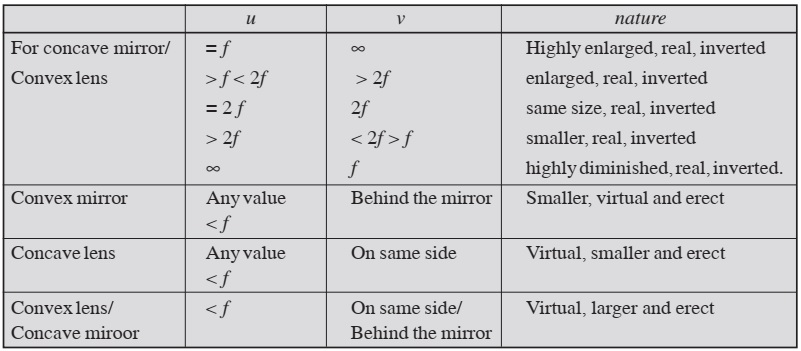# Optics: Light Energy

Light is the form of energy which makes the objects visible to us. When light reaches from object to our eyes, it becomes visible to us. Reflection, refraction and dispersion are the important properties of light.

In reflection the angle of incidence is equal to the angle of reflection and the frequency, speed and wavelength of light remain unchanged.

In refraction, the ratio of the speed of light in one medium to another medium remains constant and called refractive index. Refractive index of medium 2 with respect to medium 1 is,

n12 = speed of light in medium 1 / speed of light in medium 2

The refractive index is also measured as the ratio of sine of angle of incidence to sine of angle of refraction.

n = sin i / sin r

For spherical mirror, the focal length of the mirror is half of the radius of curvature. The focal length (f), object distance (u) and image distance (v) for the mirror are related as

1/f = 1/v + 1/u

This relation is called mirror formula. The distances referred to are always measured from the pole. The distances measure in the direction of incident ray are taken as positive and in opposite direction of incident ray are taken as negative.

In plane mirror virtual image of same size as object is formed behind the mirror at a distance equal to the distance of the object from the mirror. Due to reflection in plane mirror left handedness is changed into right handedness and vice-versa. This is known as lateral inversion. However, the mirror does not invert vertically up and down.

In refraction the image formed appears little closer as compared to the distance of real object such that

apparent depth = Real depth / Refractive index

The focal length of lens (f), the distance of object (u) and distance of image (v) from the lens are related as:

1/f = 1/v - 1/u

This relation is called lens formula.When light passes through a prism it splits into its constituents colours. This phenomenon is called dispersion of light. The rainbow is the consequence of dispersion of light.

A person who can see distant objects and not able to see the nearby objects is suffering from hypermetropia or long-sightedness. This defect of vision can be corrected by using convex lens. A person who can see the nearby objects and not able to see the distant objects is suffering from myopia or short-sightedness. This defect of vision can be removed by using concave lens.

The least distance of distinct vision for normal human eye is 25 cm and far distance is infinity.